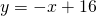# Making y=y

When two lines are given in slope intercept form, it is a simple enough procedure to substitute the second expression forinto the first equation.

Example:

Suppose we are looking for the one ordered pair that satisfies both equations:We are looking for an ordered pair (x, y) that satisfies both equations – that is, when we find the solution, thecoordinate is the same for both equations. The condition that we use to solve this system is that for some value,That is, instead of writingon the left, we substituted the other expression for. Now we have a reasonably straightforward linear equation to solve.

Upon solving we have:We now replace thewithon either original equation to find. Usingwe have.

Solution:,.

On the first applet, take the second expression forand substitute it into the first equation, to find if there is ancoordinate that yields the samecoordinate on both lines. Remember that for coincident lines, there are infinitely many solutions; and for parallel lines, there are is no solution.

# Substitute into the middle of an expression

On the second applet, one of the equations is given in general form. Take the expression forin the second equation and substitute it into the first to find the solutionthat yields the sameon both lines.

Example:Solution:,.

# Substituting when neither variable is the subject of either equation

Rearrange one or the other of the equations to make eitherorthe subject. Then substitute into the other equation to find one part of the solution. Complete by finding the second part. Enter the values into the x, y input boxes to check the solution and to see the graphical representation of the solution.